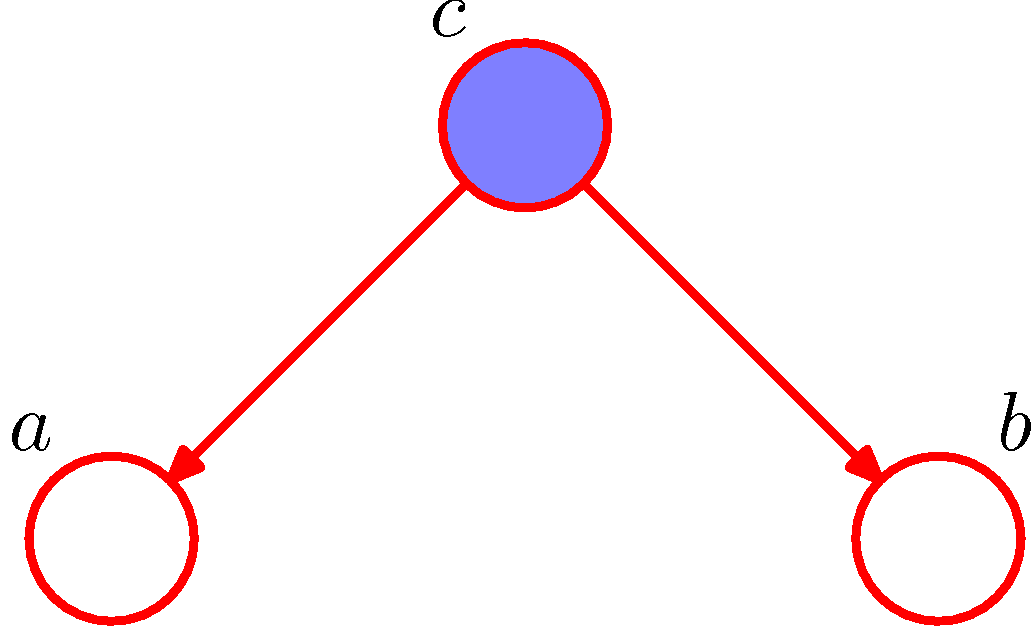# [Probability] d-Separation

#### Serial Connectionsp(a,b) = p(a) sum_c p(c|a)p(b|c) = p(a)p(b|a) ~= p(a)p(b), so a and b are not marginally independent (causal chain)

if c is given,

p(a,b|c) = p(a,b,c)/p(c) = p(a)p(c|a)p(b|c)/p(c) = p(a|c)p(b|c), so a and b are conditionally independent given c (d-Separable by case I)

#### Diverging Connectionsp(a,b) = sum_c p(a|c)p(b|c)p(c) ~= p(a)p(b), so a and b are not marginally independent (common cause)

if c is given,
p(a,b|c) = p(a,b,c)/p(c) = p(a|c)p(b|c), so a and b are conditionally independent given c (d-Separable by case II)

#### Converging Connections

p(a,b) = p(a)p(b), so a and b are marginally independent (unobserved common effect)

if c is given,

p(a,b|c) = p(a,b,c)/p(c) = p(a)p(b)p(c|a,b)/p(c) ~= p(a|c)p(b|c), so a and b are conditionally dependent given c

Also, if any of c’s child is given, same applies. (d-Separable by case III)

#### Reference

This site uses Akismet to reduce spam. Learn how your comment data is processed.